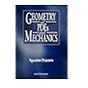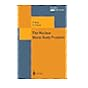Normal view MARC view ISBD view

Principles of advanced mathematical physics. Volume II.530.1 POK Gauge field theories. 530.1 PRA Geometry of PDEs and mechanics. 530.1 REC Recent developments in mathematical physics; 530.1 RIC Principles of advanced mathematical physics. Volume II. 530.1 RIC Principles of advanced mathematical physics. Volume I. 530.1 RIN The nuclear many-body problem / 530.1 ROE Path integral approach to quantum physics - an introduction.